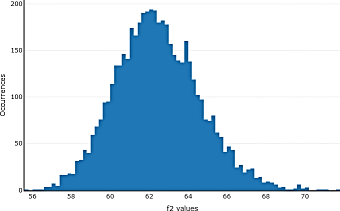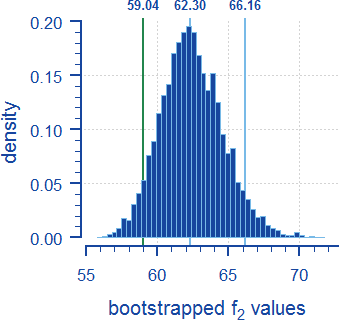## bootf2BCA v1.1 [Software]

Dear all,

we discussed the code at BioBridges…

The problem with reproducibility can easily be solved.
In the script F2_boot.R after line 270 #main algorithm add a new line

set.seed(X)

where X is an integer (specified in the protocol and report). Use

c(-1L, +1L) * .Machine\$integer.max

to check the possible range. On my machine it gives

 -2147483647  2147483647

However, I suggest a simple one (42?).

❝ Be warned: The source code is hard to read, nearly no comments at all.

The current version 1.2 is a little bit better. Still heavy stuff.

The cut-off preferred by the EMA is implemented:Don’t get confused by Q>=85%. That’s typo. Actually it is the correct >85%.
Still missing: One value >85% for the test (FDA) or one value >85% for the comparator (WHO).

❝ Be further warned: If you rise the number of bootstrap samples (default is 1000, too low IMHO) the result file will contain a huge number of entries, each bootstrap sample is recorded! The same mess as in the 'Object Pascal' implementation. But could of course also changed with a little knowledge of R.

Hint: In F2_boot.R comment out the loop in lines 317–329. Then the report-file shrinks from a couple of megabytes to some kilobytes. I would keep the rest for documentation. Useful to read the bootstrapped ƒ2 values from the file and generate your own histogram (density instead of counts). I don’t like the one ofplotly. Way too many bins for my taste (here an example with 5,000 bootstraps). $$\small{\textrm{ceiling}(\sqrt n)}$$ gives 71 bins and smells of Excel.I prefer the Freed­man–Dia­conis rule* and show additionally the median and 90% CI (green line if the lower CL ≥50).• Even if you specify hist(..., breaks="Freed­man–Dia­conis") this is not what you might expect from the Freedman–Diaconis rule $$width=2\frac{\textrm{IQR}(x)}{\sqrt{n}}$$ and $$bins=[\textrm{max}(x)-\textrm{min}(x)]/width$$.
The man page ofhist states:
[…] the number is a suggestion only; as the breakpoints will be set topretty values.
In my example I got 34 bins instead of the desired 47 (IQR=2.9026, n=5,000, min=55.76, max=71.73). Workaround (x are the bootstrapped ƒ2-values):
  width <- 2*IQR(x)/length(x)^(1/3)   bins  <- as.integer(diff(range(x)) / width)   hist(x, breaks = seq(min(x), max(x), length.out = bins), freq = FALSE)

Dif-tor heh smusma 🖖🏼 Довге життя Україна!Helmut SchützThe quality of responses received is directly proportional to the quality of the question asked. 🚮
Science QuotesIng. Helmut Schütz